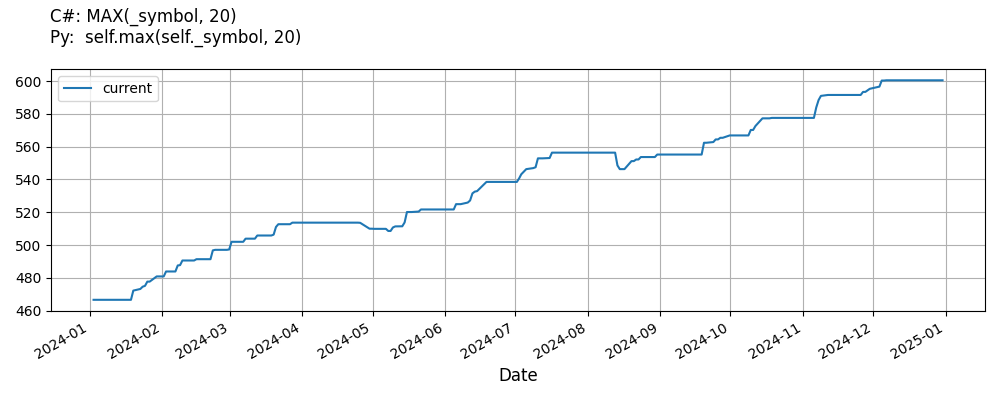# Supported Indicators

## Maximum

### Introduction

This indicator represents an indicator capable of tracking the maximum value and how many periods ago it occurred

To view the implementation of this indicator, see the LEAN GitHub repository.

### Using MAX Indicator

To create an automatic indicators for Maximum, call the MAX helper method from the QCAlgorithm class. The MAX method creates a Maximum object, hooks it up for automatic updates, and returns it so you can used it in your algorithm. In most cases, you should call the helper method in the Initialize method.

public class MaximumAlgorithm : QCAlgorithm
{
private Symbol _symbol;
private Maximum _max;

public override void Initialize()
{
_max = MAX(_symbol, 20);
}

public override void OnData(Slice data)
{
{
// The current value of _max is represented by itself (_max)
// or _max.Current.Value
Plot("Maximum", "max", _max);
// Plot all properties of max
Plot("Maximum", "periodssincemaximum", _max.PeriodsSinceMaximum);
}
}
}
class MaximumAlgorithm(QCAlgorithm):
def Initialize(self) -> None:
self.max = self.MAX(self.symbol, 20)

def OnData(self, slice: Slice) -> None:
# The current value of self.max is represented by self.max.Current.Value
self.Plot("Maximum", "max", self.max.Current.Value)
# Plot all attributes of self.max
self.Plot("Maximum", "periodssincemaximum", self.max.PeriodsSinceMaximum)


The following reference table describes the MAX method:

### MAX()1/1

            Maximum QuantConnect.Algorithm.QCAlgorithm.MAX (
Symbol                           symbol,
Int32                            period,
*Nullable<Resolution>      resolution,
*Func<IBaseData, Decimal>  selector
)


Creates a new Maximum indicator to compute the maximum value.

If you don't provide a resolution, it defaults to the security resolution. If you provide a resolution, it must be greater than or equal to the resolution of the security. For instance, if you subscribe to hourly data for a security, you should update its indicator with data that spans 1 hour or longer.

You can manually create a Maximum indicator, so it doesn't automatically update. Manual indicators let you update their values with any data you choose.

Updating your indicator manually enables you to control when the indicator is updated and what data you use to update it. To manually update the indicator, call the Update method with time/number pair, or an IndicatorDataPoint. The indicator will only be ready after you prime it with enough data.

public class MaximumAlgorithm : QCAlgorithm
{
private Symbol _symbol;
private Maximum _max;

public override void Initialize()
{
_max = new Maximum(20);
}

public override void OnData(Slice data)
{
if (data.Bars.TryGeValue(_symbol, out var bar))
{
_max.Update(bar.EndTime, bar.Close);
}

{
// The current value of _max is represented by itself (_max)
// or _max.Current.Value
Plot("Maximum", "max", _max);
// Plot all properties of max
Plot("Maximum", "periodssincemaximum", _max.PeriodsSinceMaximum);
}
}
}
class MaximumAlgorithm(QCAlgorithm):
def Initialize(self) -> None:
self.max = Maximum(20)

def OnData(self, slice: Slice) -> None:
bar = slice.Bars.get(self.symbol)
if bar:
self.max.Update(bar.EndTime, bar.Close)

# The current value of self.max is represented by self.max.Current.Value
self.Plot("Maximum", "max", self.max.Current.Value)
# Plot all attributes of self.max
self.Plot("Maximum", "periodssincemaximum", self.max.PeriodsSinceMaximum)


To register a manual indicator for automatic updates with the security data, call the RegisterIndicator method.

public class MaximumAlgorithm : QCAlgorithm
{
private Symbol _symbol;
private Maximum _max;

public override void Initialize()
{
_max = new Maximum(20);
RegisterIndicator(_symbol, _max, Resolution.Daily);
}

public override void OnData(Slice data)
{
{
// The current value of _max is represented by itself (_max)
// or _max.Current.Value
Plot("Maximum", "max", _max);

}
}
}
class MaximumAlgorithm(QCAlgorithm):
def Initialize(self) -> None:
self.max = Maximum(20)
self.RegisterIndicator(self.symbol, self.max, Resolution.Daily)

def OnData(self, slice: Slice) -> None:
# The current value of self.max is represented by self.max.Current.Value
self.Plot("Maximum", "max", self.max.Current.Value)



The following reference table describes the Maximum constructor:

### Maximum()1/2

            Maximum QuantConnect.Indicators.Maximum (
int  period
)


Creates a new Maximum indicator with the specified period.

### Maximum()2/2

            Maximum QuantConnect.Indicators.Maximum (
string  name,
int     period
)


Creates a new Maximum indicator with the specified period.

### Visualization

The following image shows plot values of selected properties of Maximum using the plotly library.You can also see our Videos. You can also get in touch with us via Discord.